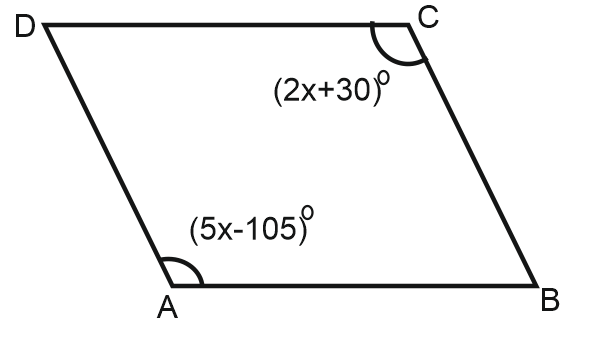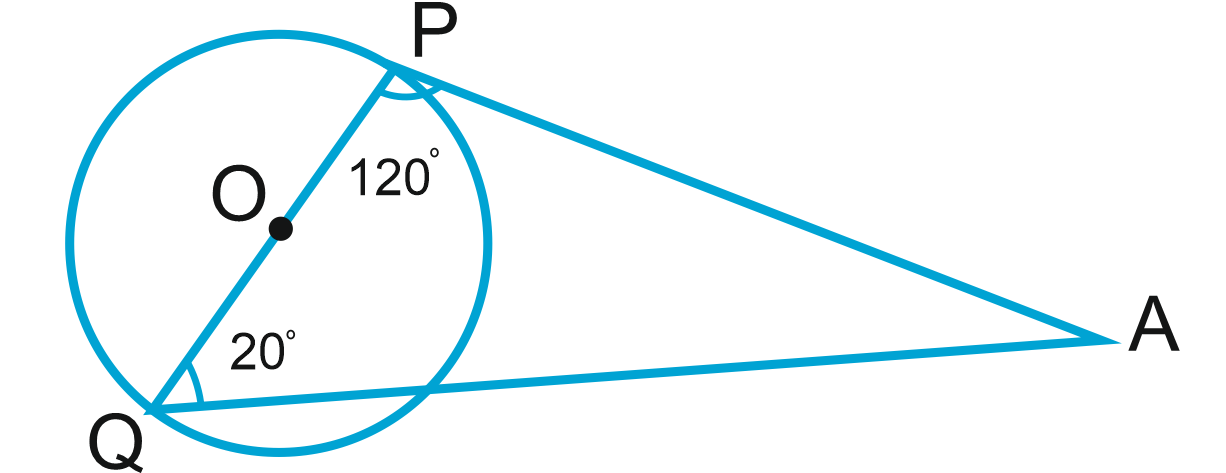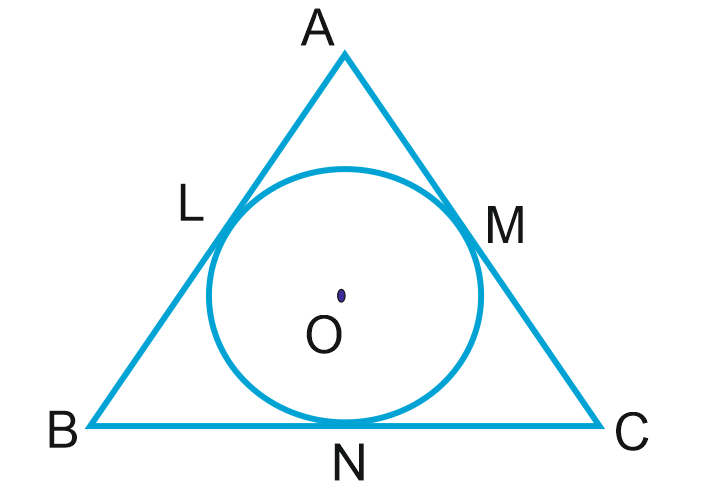• Save

# Geometry Questions for Competitive Exams

2 months ago 1.6K ViewsDear Students, As you know that Geometry is an important part of maths. Most of the students getting confused while solving questions/equations related to geometry. So, here I am sharing the latest and important questions of geometry from which you can clear your doubts and confusions.
These questions will help you to improve your score by simply cover the different types of Geometry questions. For more Practice visits on Test Series.

## Geometry Questions

Q :

In the given figure ABCD is a Parallelogram then the find out of the value of x is?(A) 25

(B) 60

(C) 75

(D) 45

Q :

In the given Figure ∠PQA = 20° and ∠APQ = 120° then the find out the ∠ PAQ=?(A) 120 degree

(B) 20 degree

(C) 40 degree

(D) 60 degree

Q :

In the given figure AB, BC and CA is a tangent of a circle if BC=6.3 cm and MC=2.7 cm then the Value of BL is(A) 3.5 cm

(B) 2.5 cm

(C) 3.6 cm

(D) 2 cm

Q :

When a diagonal of a square is 24 cm then find out of its perimeter.

(A) 28 cm

(B) 48√2 cm

(C) 36 √2 cm

(D) 46 √2 cm

Q :

If the graph of the equations x + y = 0 and 5y + 7x =24 intersect at (m, n), then the value of m + n is :

(A) 2

(B) 1

(C) 0

(D) – 1

Q :

Equation of the straight line parallel to x-axis and also 3 units below x-axis is:

(A) x = - 3

(B) y = 3

(C) y = -3

(D) x = 3

Q :

The midpoints of AB and AC of a triangle ABC are X and Y respectively. If BC + XY = 12 units, then BC – XY is:

(A) 10 units

(B) 8 units

(C) 6 units

(D) 4 units